# Martin's Blog

## Absolute Hodge classes in l-adic cohomology

Posted by Martin Orr on Friday, 26 June 2015 at 11:30

We can define absolute Hodge classes in-adic cohomology in the same way as absolute Hodge classes in de Rham cohomology. We can then prove Deligne's theorem, that Hodge classes on an abelian variety are absolute Hodge, for-adic cohomology. Because it is easy to prove that absolute Hodge classes in-adic cohomology are potentially Tate classes, this implies half of the Mumford-Tate conjecture.

In particular, it implies that if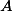is an abelian variety over a number field, then a finite index subgroup of the image of the-adic Galois representation onis contained in the-points of the Mumford-Tate group of. This is the goal I have been working towards for some time on this blog.

Deligne's definition of absolute Hodge classes considered-adic cohomology (for all) and de Rham cohomology simultaneously. The accounts I read of this theory focussed on the de Rham side, leading me to believe that the de Rham part was essential and the-adic part an optional extra. This is why I wrote the past few posts about de Rham cohomology and am now adding the-adic version on at the end, even though I am more interested in the-adic version. Now that I understand what is going on, I realise that I could have used only-adic cohomology from the beginning. One day I might write up a neater account which uses-adic cohomology only.

Before the main part of this post, talking about absolute Hodge classes in-adic cohomology, I need to talk about Tate twists in-adic cohomology. These are more significant than Tate twists in singular cohomology because they change the Galois representations involved. This resulted in some mistakes in my previous posts on Tate classes, which I think I have now fixed.

Read more...

## Deligne's Principle A and the Mumford-Tate conjecture

Posted by Martin Orr on Wednesday, 10 September 2014 at 11:20

In this post I will fill in a missing detail from two weeks ago, where I mentioned that the Mumford-Tate group is determined by the Hodge classes. More precisely, I will show that an elementof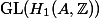is in the Mumford-Tate group if and only if every Hodge class on every Cartesian power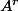is an eigenvector of. In the context of Deligne's theorem on absolute Hodge classes, this is known as Principle A.

We will also see that a version of this statement holds for the-adic monodromy group and Tate classes. This implies a link between the Hodge, Tate and Mumford-Tate conjectures.

Read more...

## Tate classes

Posted by Martin Orr on Tuesday, 02 September 2014 at 19:30

In my last post I talked about Hodge classes on abelian varieties. Today I will talk about the analogue in-adic cohomology, called Tate classes. Tate classes are defined to be classes in a Tate twist of the-adic cohomology on which the absolute Galois group of the base field acts trivially.

The Tate classes on a variety change if we extend the base field (because this changes the Galois group). They are mainly interesting in the case in which the base field is finitely generated. In this post I will also define potentially Tate classes, which depend less strongly on the base field (they are unchanged by finite extensions).

I will state the Tate conjecture, the-adic analogue of the Hodge conjecture, which says that if the base field is finitely generated, then the vector space of Tate classes is spanned by classes of algebraic cycles. I will also mention some other conjectures which are implied by or equivalent to the Tate conjecture or a slight strengthening of it.

Unlike in the case of Hodge classes, we cannot easily ignore the Tate twist in the definition of Tate classes. This post only contains brief remarks on Tate twists; there is a link to a later post with a more detailed discussion.

Read more...

## Main steps of the proof of the period theorem

Posted by Martin Orr on Friday, 03 August 2012 at 11:06

Today I will explain how to prove the Masser-Wüstholz Period Theorem starting from the Key Proposition, an weaker existence result for abelian subvarieties of bounded degrees. The Key Proposition and the Tangent Space Lemma, which I mention briefly, are the main results proved by Masser and Wüstholz by the techniques of transcendental number theory on their way to the Period Theorem.

Recall that the Period Theorem is a bound for the degree of the smallest abelian subvariety ofwhose tangent space contains a given period. In the Key Proposition we find a subvariety of bounded degree whose tangent space satisfies the very weak condition of being inside a chosen hyperplane in. In particularneed not be in the tangent space of the subvariety. However we use the Key Proposition and induction to prove the Strong Proposition, which gives a subvariety whose tangent space does contain. Several applications of the Strong Proposition with different hyperplanes inprove the Period Theorem.

Read more...

## The matrix lemma for elliptic curves

Posted by Martin Orr on Friday, 25 May 2012 at 14:00

Letbe a principally polarised abelian variety of dimensionover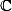. We can associate withacomplex matrix called the period matrix which roughly speaking describes a basis for the image of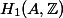in(actually it is not really the period matrix as it is only defined up to the action ofon the Siegel upper half space; we can make it nearly unique by forcing it to be in a particular fundamental domain).

The matrix lemma says that, ifis defined over a number field, then the entries of the imaginary part of the period matrix cannot be too large with respect to the height of(Faltings height or modular height).

Matrix lemma. (Masser 1987) Letbe a principally polarised abelian variety of dimensionover a number field. Letbe the period matrix forin the standard fundamental domain of the Siegel upper half space. There is a constantdepending only onsuch that all the entries ofsatisfy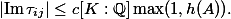Last time I used a lower bound for the lengths of non-zero periods in the proof of the isogeny theorem. This follows from the matrix lemma as we can easily relate lengths of periods and the period matrix.

Today I will prove the matrix lemma for elliptic curves. The general proof requires various facts about Siegel modular forms and also uses a funny choice of level structure due to Igusa (I do not understand why). But the basic structure of the proof is already visible in the elliptic curves case and we can be concrete about the modular forms involved, using only facts I learned in Part III.

Read more...